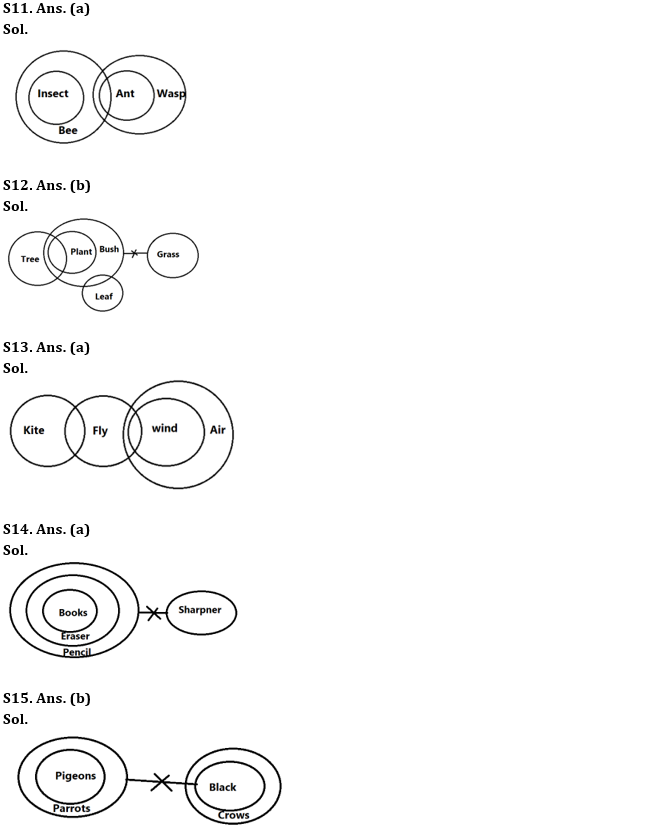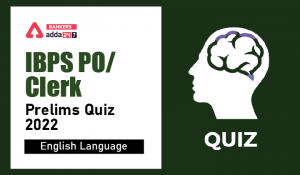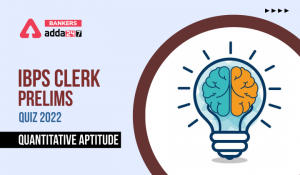Latest Banking jobs   »

# Reasoning Ability Quiz For IBPS Clerk Prelims 2022- 26th July

Directions (1-5): Study the following arrangement of numbers & symbols carefully & answer the following questions.

7 @ # 6 7 \$ 1 2 % 3 4 8 ! 3 \$ 7 6 & * 4 9 0 ^ 6 7 & 5

Q1. How many symbols are immediately preceded and immediately followed by odd number?
(a) One
(b) Two
(c) Three
(d) Four
(e) None of the above

Q2. What will be the sum of all even numbers in the given series between seventh element from left end and third element from right end?
(a) 30
(b) 28
(c) 32
(d) 34
(e) None of the above

Q3. Which of the following element is tenth to the left of tenth element from right end?
(a) 7
(b) \$
(c) 1
(d) 2
(e) None of these

Q4. What will be the sum of all prime numbers in the given series between fifth element from left end and ninth element from right end?
(a) 14
(b) 15
(c) 16
(d) 17
(e) None of the above

Q5. How many even numbers are immediately preceded by odd number and immediately followed by even number?
(a) One
(b) Two
(c) Three
(d) Four
(e) None of the above

Directions (6-10): Study the given numeric series and answer the questions based on it.

512 625 962 348 245

Q6. If the position of first and third digit within the number are interchanged, which of the following will become the third highest number?
(a) 962
(b) 625
(c) 512
(d) 245
(e) 348

Q7. If all the three digits are arranged in ascending order within the number, which of the following will be second lowest number?
(a) 962
(b) 625
(c) 512
(d) 245
(e) 348

Q8. If in each number, the first two digits are multiplied, which number will give the smallest resultant?
(a) 962
(b) 625
(c) 512
(d) 245
(e) 348

Q9. If the positions of first and second digit is interchanged within the number, and one is added to the last digit of each of the numbers, then how many numbers thus formed will be odd numbers?
(a) None
(b) One
(c) Two
(d) Three
(e) None of these

Q10. What will be the resultant when the third digit of the lowest number and the second digit of the highest number are multiplied?
(a) 45
(b) 30
(c) 40
(d) 54
(e) None of these

Directions (11-15): In the question below, three statements are given followed by the conclusions. You have to take the given statements to be true even if they seem to be at variance with commonly known facts. Read all the conclusions and then decide which of the given conclusions logically follows from the given statements disregarding commonly known facts.

Q11. Statements: Only bee is insects.
Some bees are ants.
Each ant is wasp.
Conclusions: I. All insects are bees.
II. Some wasp is insects.
(a) Only conclusion I follows
(b) Only conclusion II follows
(c) Either conclusion I or II follows
(d) Both conclusions I and II follow
(e) Neither conclusion I nor II follows

Q12. Statements: Some plants are trees.
All plants are bush.
No bush is grass.
Some bush is leaf.
Conclusions: I. Some tree is leaf.
II. No plant is grass
(a) Only conclusion I follows
(b) Only conclusion II follows
(c) Either conclusion I or II follows
(d) Both conclusions I and II follow
(e) Neither conclusion I nor II follows

Q13. Statements: Some kite are fly.
Some fly are wind.
All wind are air.
Conclusions: I. Some fly are air.
II. Some kite are wind.
(a) Only conclusion I follows
(b) Only conclusion II follows
(c) Either conclusion I or II follows
(d) Both conclusions I and II follow
(e) Neither conclusion I nor II follows

Q14. Statements: All Erasers is pencil.
All books are Erasers.
No sharpner is pencil.
Conclusions: I. Some eraser can never be a sharpner.
II. All those erasers which are pencil are sharpner is a possibility.
(a) Only conclusion I follows
(b) Only conclusion II follows
(c) Either conclusion I or II follows
(d) Both conclusions I and II follow
(e) Neither conclusion I nor II follows

Q15. Statements: All pigeons are parrot.
No parrot is black.
All blacks are Crows.
Conclusions: I. Some pigeons being black is a possibility.
II. At least some parrots are pigeons.
(a) Only conclusion I follows
(b) Only conclusion II follows
(c) Either conclusion I or II follows
(d) Both conclusions I and II follow
(e) Neither conclusion I nor II follows

Solutions

S1. Ans. (c)
Sol. Three – 7 \$ 1, 3 \$ 7, 7 & 5

S2. Ans. (a)
Sol. Required sum = 2 + 4 + 8 + 6 + 4 + 6 = 30.

S3. Ans. (d)
Sol. Tenth element from right end – &; So, tenth element to the left of & – 2

S4. Ans. (b)
Sol. Required sum = 2 + 3 + 3 + 7 = 15

S5. Ans. (a)
Sol. One – 3 4 8

S6. Ans. (b)
Sol. After operation – 215 526 269 843 542
So, third highest number = 526. Hence, 625 is the correct answer.

S7. Ans. (d)
Sol. After operation – 125 256 269 348 245
So, second lowest number = 245. Hence, 245 is the correct answer.

S8. Ans. (c)
Sol. After multiplication of 1st and 2nd digit in each number, 512 will give the smallest resultant.

S9. Ans. (d)
Sol. After operation – 152 265 692 438 425
New series – 153 266 693 439 426; So, there are three odd numbers.

S10. Ans. (b)
Sol. Third digit of lowest number – 5, Second digit of highest number – 6
Required multiplication = 5 x 6 = 30.#### Congratulations!Incorrect details? Fill the form again here

•English Quizzes For IBPS Clerk/PO Prelim...
•English Quizzes For IBPS Clerk/PO Prelim...
•Quantitative Aptitude Quiz For IBPS Cler...
•Quantitative Aptitude Quiz For IBPS Cler...
•Reasoning Ability Quiz For IBPS Clerk/PO...
•Quantitative Aptitude Quiz For IBPS Cler...